Chapter Notes: Surface Area & Volumes

# Surface Area & Volumes Chapter Notes - Mathematics (Maths) Class 10

 Table of contents1. Surface Area of a Combination of Solids(i) Surface Area of a Cube(ii) Surface Area of a Cuboid(iii) Surface Area of a Right Circular Cylinder(iv) Surface Area of a Hollow Right Circular Cylinder(v) Surface Area of a Right Circular Cone(vi) Surface Area of a Sphere(vii) Surface Area of a Hemisphere2. Volume of Combination of Solids(i) Volume of Cube(ii) Volume of Cylinder(iii) Volume of Cone(iv) Volume of Sphere(v) Volume of Hemi-SphereThree dimensions can be measured, length, width, and height, for any object that you can see or touch. There are certain dimensions of our home that we live in. The rectangular display screen/Monitor you’re looking at has the width and breadth of its own length.
For every three-dimensional geometrical structure, surface area and volume are measured.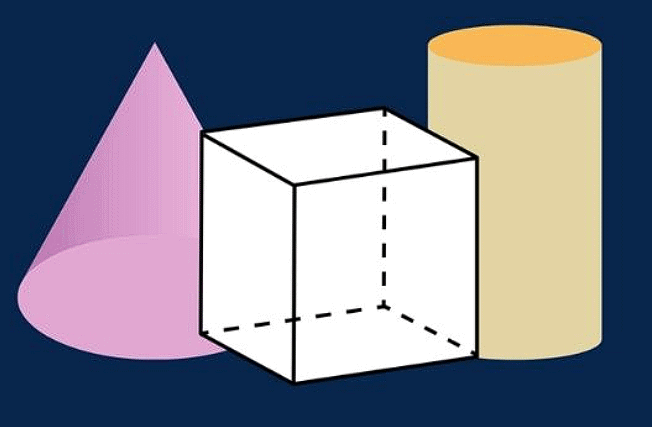Surface Area & Volume

## 1. Surface Area of a Combination of Solids

Surface area refers to the measurement of the space taken up by a flat two-dimensional surface. This measurement is expressed in terms of square units. For three-dimensional objects, the surface area represents the space occupied by their outer surfaces. Similar to the flat surface area, it is also measured in square units.
In general, there are two types of surface area

### (a) Total Surface Area

Total surface area is the combined measurement of the base(s) and the curved portion of an object. It represents the sum of the object's surface coverage. In cases where the object's shape includes both a curved surface and a base, the total surface area is calculated by summing up the measurements of these two areas.

### (b) Curved Surface Area or Lateral Surface Area

Curved surface area pertains to the measurement of only the curved portion of a shape, excluding any base(s). This aspect is also referred to as the lateral surface area, particularly for shapes like a cylinder.

Question for Chapter Notes: Surface Area & Volumes
Try yourself:What does the "Total Surface Area" of an object include?

## (i) Surface Area of a Cube

Cube is a solid shape having 6 equal square faces of length l.

Lateral surface area of a cube =4l2

Total surface area of cube=6l 2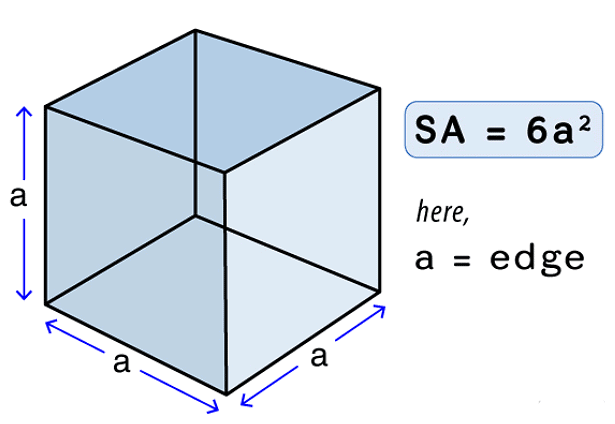(ii) Surface Area of a Cuboid

Cuboid is a solid shape having 6 rectangular faces at a right angle with length l, breadth b and height h.

Lateral surface area of a cuboid= 2h(l+b)

Total surface area of a cuboid= 2(lb+bh+lh)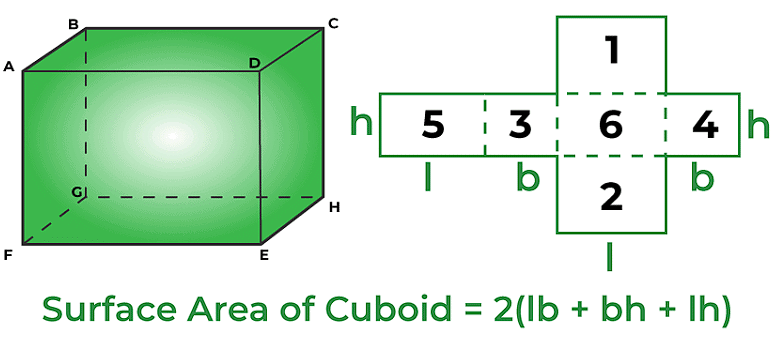## (iii) Surface Area of a Right Circular Cylinder

If we fold a rectangular sheet with one side as its axis then it forms a cylinder. It is the curved surface of the cylinder. And if this curved surface is covered by two parallel circular bases then it forms a right circular cylinder. For a cylinder of height h and with base radius r.

Curved surface area of a Right circular cylinder=2πrh

Total surface area of a Right circular cylinder=2πr2+2πrh=2πr(r+h)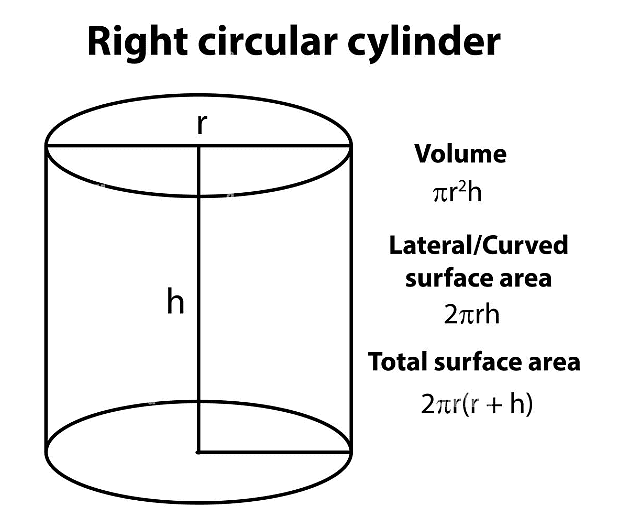Question for Chapter Notes: Surface Area & Volumes
Try yourself:What is the formula for the total surface area of a cuboid with length 'l', breadth 'b', and height 'h'?

## (iv) Surface Area of a Hollow Right Circular Cylinder

If a right circular cylinder is hollow from inside then it has a different curved surface.

Curved surface area of a Right circular cylinder=2πh(R+r)

Total surface area of a Right circular cylinder=2πh(R+r)+2π(R2 −r2)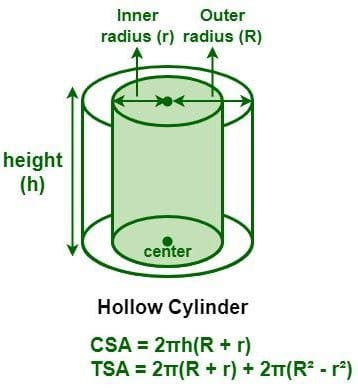## (v) Surface Area of a Right Circular Cone

If we revolve a right-angled triangle about one of its sides by taking other as its axis then the solid shape formed is known as a Right Circular Cone.

Curved surface area of a Right Circular Cone = πrl=πrh2+r2

Total surface area of a Right Circular Cone= πr2+πrl=πr(r+l)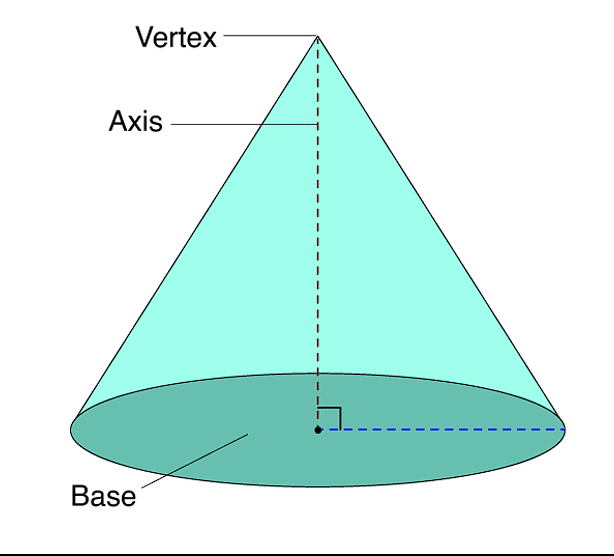## (vi) Surface Area of a Sphere

A sphere is a solid shape which is completely round like a ball. It has the same curved and total surface area.
Curved or Lateral surface area of a Sphere=4πr2

Total surface area of a Sphere=4πr2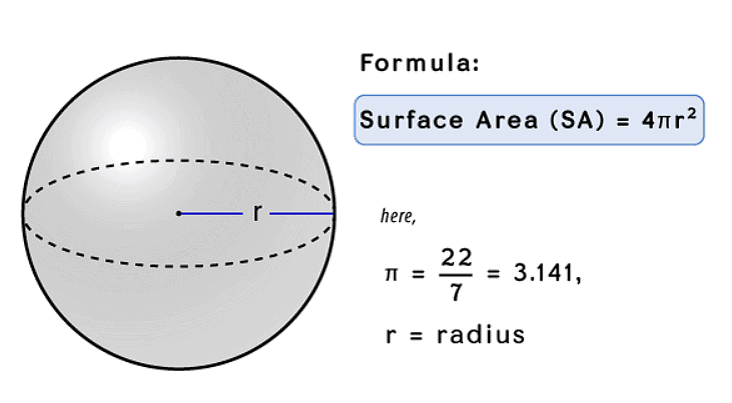## (vii) Surface Area of a Hemisphere

If we cut the sphere in two parts then it is said to be a hemisphere.

Curved or Lateral surface area of a Hemisphere=2πr2

Total surface area of a Hemisphere=3πr2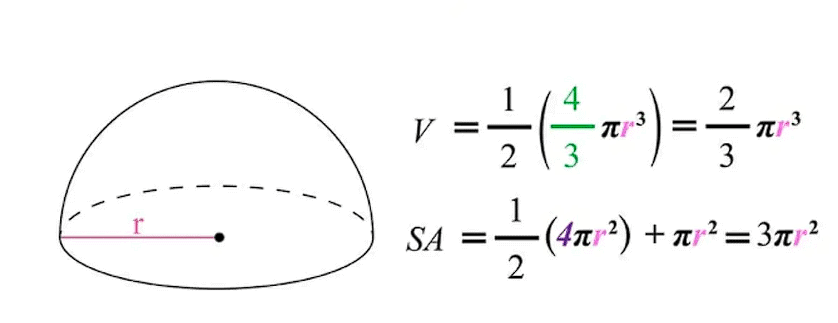Question for Chapter Notes: Surface Area & Volumes
Try yourself:What is the total surface area of a sphere with radius 'r'?

## 2. Volume of Combination of Solids

The number of cubic units needed to fill a solid figure. We will learn how to figure out the volume of cubes and rectangular prisms.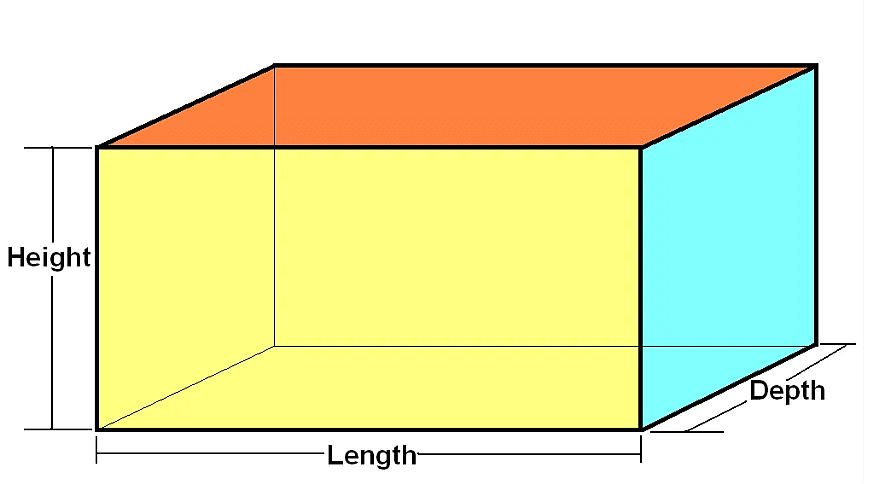• Curved/lateral Surface Area = 2h(l +b)
• Surface Area = (lb+bh+lh)
• Volume = lbh

## (i) Volume of Cube

Side of cube = a

• Curved/lateral surface area = 4a2
• Total Surface Area = 6a2
• Volume = a3

## (ii) Volume of Cylinder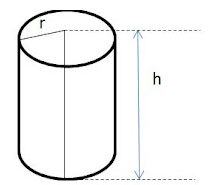h = Height of Cylinder

• Curved Surface Area = 2πrh
• Total Surface Area = 2πrh +2 πr2
• Volume = πr2h

Question for Chapter Notes: Surface Area & Volumes
Try yourself:What is the formula for the volume of a cylinder with radius 'r' and height 'h'?

## (iii) Volume of Cone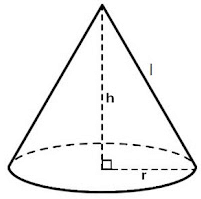h= height of cone

l = slant height of cone = (h2+r2)

• Curved Surface Area = πrl
• Total Surface Area = πrl + πr2h
• Volume = 1/3πr2h

## (iv) Volume of Sphere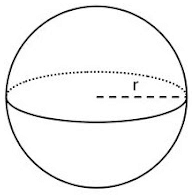• Volume =(V)= 4/3πr3

## (v) Volume of Hemi-Sphere

• Volume =4/3πr3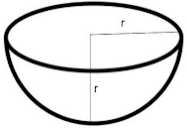• Curved Surface Area = πr2
• Total Surface Area = 2πr2
• Volume = 2/3πr3
The document Surface Area & Volumes Chapter Notes | Mathematics (Maths) Class 10 is a part of the Class 10 Course Mathematics (Maths) Class 10.
All you need of Class 10 at this link: Class 10

## Mathematics (Maths) Class 10

115 videos|478 docs|129 tests

## FAQs on Surface Area & Volumes Chapter Notes - Mathematics (Maths) Class 10

 1. What is the formula for calculating the surface area of a cylinder?Ans. The formula for calculating the surface area of a cylinder is 2πr(r+h), where r is the radius of the base and h is the height of the cylinder.
 2. How do you calculate the volume of a sphere?Ans. The volume of a sphere can be calculated using the formula (4/3)πr^3, where r is the radius of the sphere.
 3. Can you explain how to find the surface area of a cone?Ans. To find the surface area of a cone, you can use the formula πr(r + l), where r is the radius of the base and l is the slant height of the cone. The slant height can be found using the Pythagorean theorem.
 4. What is the formula for finding the volume of a rectangular prism?Ans. The formula for finding the volume of a rectangular prism is length × width × height.
 5. How do you calculate the surface area of a cube?Ans. The surface area of a cube can be calculated by using the formula 6s^2, where s is the length of each side of the cube.

## Mathematics (Maths) Class 10

115 videos|478 docs|129 testsExplore Courses for Class 10 examSignup to see your scores go up within 7 days! Learn & Practice with 1000+ FREE Notes, Videos & Tests.
10M+ students study on EduRev
Track your progress, build streaks, highlight & save important lessons and more!
Related Searches

,

,

,

,

,

,

,

,

,

,

,

,

,

,

,

,

,

,

,

,

,

;